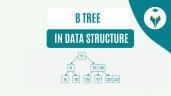Power Function in C

# Power Function in C

clickHere
Updated on Nov 28, 2023 10:57 IST

Have you ever wondered how mathematical calculations involving exponents are handled in the C programming language? One of the key functions used for this purpose is the pow() function, which is part of the math.h header file. Let’s understand more!

The Power Function is used to raise a number to the power of another number. In C Programming, this functionality is provided through the <math.h> header file and can be accessed using the pow function.

Must Read Power Function in C++ & Power Function in Java

## Syntax for the pow() function

`double pow(double base, double exponent);`

Where,

• base is the number to be raised.
• exponent is the power to which the base number is raised.

Example of Power Function in C

```#include <stdio.h>#include <math.h> int main() {    double base = 3.0;    double exponent = 4.0;        double result = pow(base, exponent);        printf("Result: %.2f\n", result);    return 0;}Copy code```

Output

`Result: 81.00`

In the code above, the base (3.0) is raised to the power of the exponent (4.0), and the result (81.00) is printed to the console. Note that the header file needs to be included in order to use the pow function, and the header file is required to use the printf function for output. The %.2f in the printf function is a format specifier that instructs the function to print the floating-point number with two decimal places.

## Examples on Power Function in C

### Example 1

Calculating the square of a number

```#include <stdio.h>#include <math.h> int main() {    double num = 4.0;    double result = pow(num, 2.0);    printf("The square of %.2f is: %.2f\n", num, result);    return 0;}Copy code```

Output

`The square of 4.00 is: 16.00`

### Example 2

Calculating the cube of a number

```#include <stdio.h>#include <math.h> int main() {    double num = 3.0;    double result = pow(num, 3.0);    printf("The cube of %.2f is: %.2f\n", num, result);    return 0;}Copy code```

Output

`The cube of 3.00 is: 27.00`

### Example 3

Calculating the Square Root Using Power Function

```#include <stdio.h>#include <math.h> int main() {    double num = 16.0;    double result = pow(num, 0.5);    printf("The square root of %.2f is: %.2f\n", num, result);    return 0;}Copy code```

Output

`The square root of 16.00 is: 4.00`User Defined Functions in C | Meaning, Types and Examples
In this blog, we will discuss about User Defined Functions in C in detail from scratch! First we will understand what are functions & then, jump into the world of...read moreFunction in C | About, Types and Examples
Have you heard of functions in the C programming language? Let's explore it, including its types and examples in detail! A function in C is a collection of statements designed...read moreTop Pattern Programs in C
Pattern programs in C are coding exercises where specific patterns or shapes are printed using loops and conditional statements.

### Example 4

Calculating the Nth Root of a Number

```#include <stdio.h>#include <math.h> int main() {    double num = 27.0;    double n = 3.0;    double result = pow(num, 1.0/n);    printf("The %.2f-th root of %.2f is: %.2f\n", n, num, result);    return 0;}Copy code```

Output

`The 3.00-th root of 27.00 is: 3.00`

### Example 5

Calculating Compound Interest

The formula for calculating compound interest over time is given by:

Where:

• A: the money accumulated after n years, including interest.
• P: the principal amount (the initial amount of money)
• r: annual interest rate (decimal)
• n: the number of times that interest is compounded per year
• t: the time in years

```#include <stdio.h>#include <math.h> int main() {    double P = 1000.0;  // Initial amount of money    double r = 0.05;    // Annual interest rate in decimal form (5%)    int n = 4;          // Interest is compounded quarterly    int t = 10;         // Time in years     double A = P * pow((1 + r/n), (n*t));    printf("The amount accumulated after %d years is: %.2f\n", t, A);    return 0;}Copy code```

Output

`The amount accumulated after 10 years is: 1643.62`

Finally, we printed the accumulated amount after the specified number of years.

## Conclusion

The Power Function in C allows developers to compute exponential values with ease. Throughout this blog, we’ve demonstrated its utility in various scenarios, from simple numeric operations to complex calculations.

clickHere

I'm Esha Gupta, a B.Tech graduate in Computer Science & Engineering, specializing in Front End Web Dev. I've interned at GeeksforGeeks & Coding Minutes, fueling my passion for crafting appealing and functional digit... Read Full Bio

## Trending Technology CoursesC Programming For Beginners - Master the C LanguageC Programming For Beginners - Master the C Languag...
UDEMY4.6C for Everyone: Programming Fundamentals
Coursera4.6C for Everyone: Programming Fundamentals
Coursera4.6Starts todayC Programming: Advanced Data Types - 5
Coursera4.4Starts todayC Programming: Using Linux Tools and Libraries - 7
Coursera4.7Starts today

## Top Picks & New Arrivals